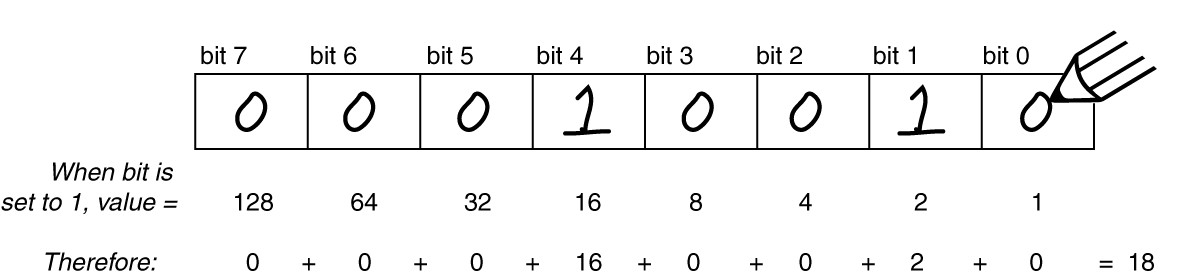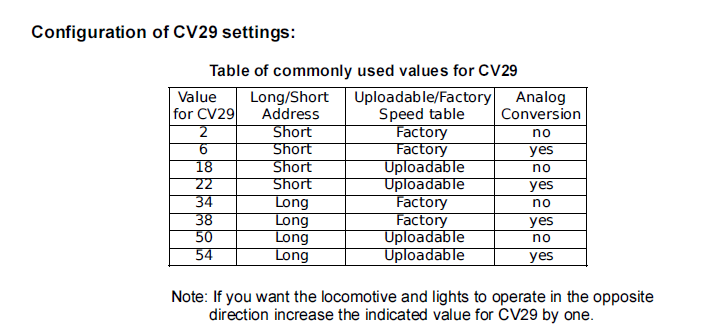Follow

# CV29 explained

use this   Handy CV29 Calculator

Ed's quick and easy tip to wrap your head around CV29: Think of it as a bank of 8 toggle switches and NOT as a real number!  CV29, each bit has a unique meaning. Here is a listing of the bit meanings:Bit 0 ON  = Direction of operation is reversed
OFF  = Direction of operation is normal
Bit 1 ON  = 28 Speed Step Mode (should always be enabled)
Bit 2 ON  = Analog mode operation enabled
OFF  = Analog mode disabled
Bit 3 is  ignored by the DCC system firmware.
Bit 4 ON  = Alternate Speed Curve Active
OFF  = Use table defined by CV 2, 5 and 6
Bit 5 ON  = Use long address in CV17/18
OFF  = Use short address defined in CV1
Bits  6 and 7 are ignored by the DCC system firmware.

Now to transfer the state of all of the individual bits as a single numeric value is where the confusing part comes into play. What is required is a little binary mathematics to calculate the final numeric value but even that is pretty easy once you know how to do it. First, start by reading a bit string from right to left. As you move to each successive bit to the left, you will notice that the bit value doubles from the previous bit value.use this   Handy CV29 Calculator